# Linear Equations Lesson 2 3 Equations l Definition

• Slides: 14Linear Equations Lesson 2. 3Equations l Definition: l l l A statement that two mathematical expressions are equal An equation always contains an equals sign = Examples The statement may be true or false. 2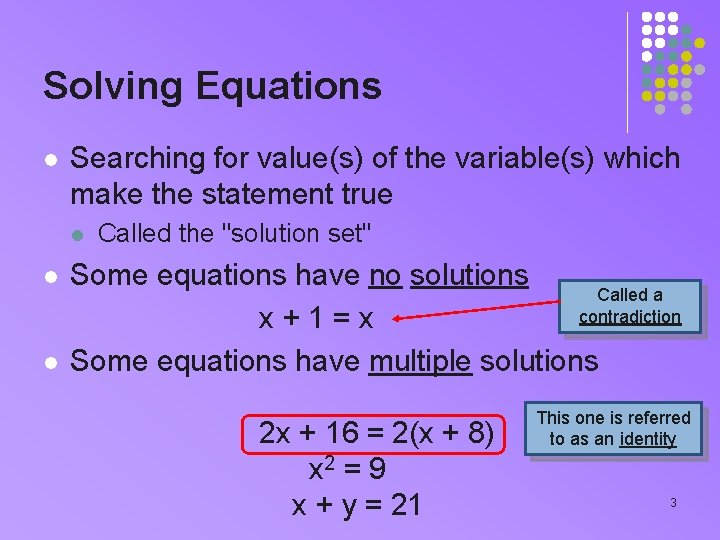Solving Equations l Searching for value(s) of the variable(s) which make the statement true l l l Called the "solution set" Some equations have no solutions Called a contradiction x+1=x Some equations have multiple solutions 2 x + 16 = 2(x + 8) x 2 = 9 x + y = 21 This one is referred to as an identity 3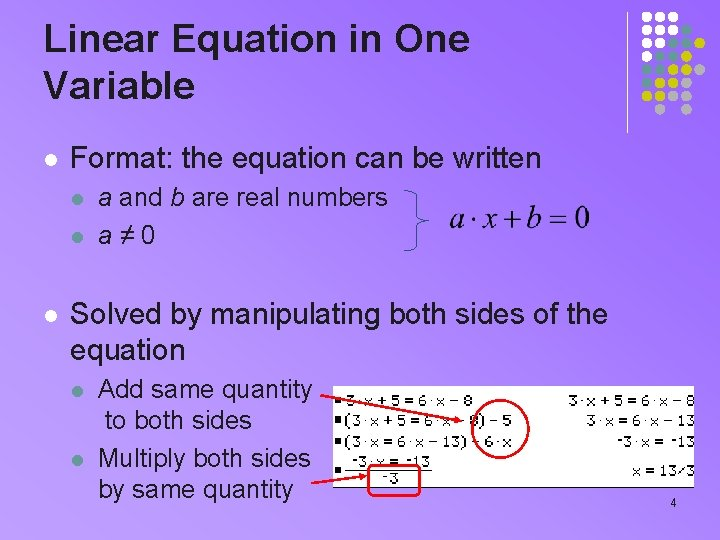Linear Equation in One Variable l Format: the equation can be written l l l a and b are real numbers a≠ 0 Solved by manipulating both sides of the equation l l Add same quantity to both sides Multiply both sides by same quantity 4Graphical Solution l Treat the equation as two separate functions l l Each side of the equals sign Specify each side as a function in the Y = screen 5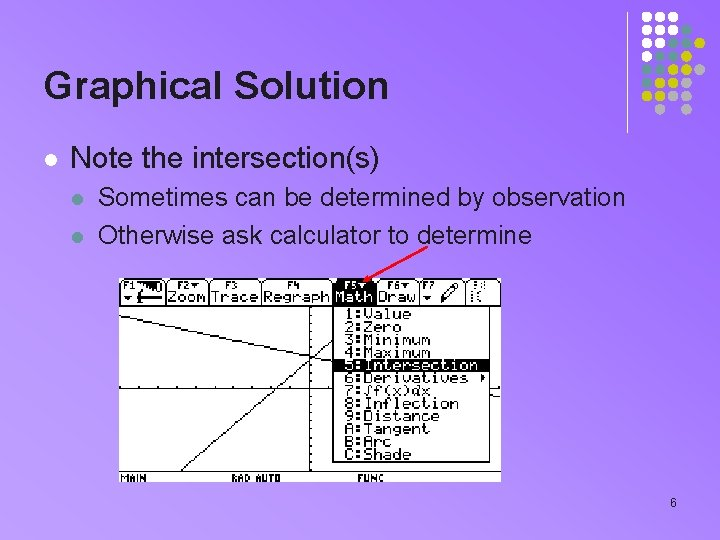Graphical Solution l Note the intersection(s) l l Sometimes can be determined by observation Otherwise ask calculator to determine 6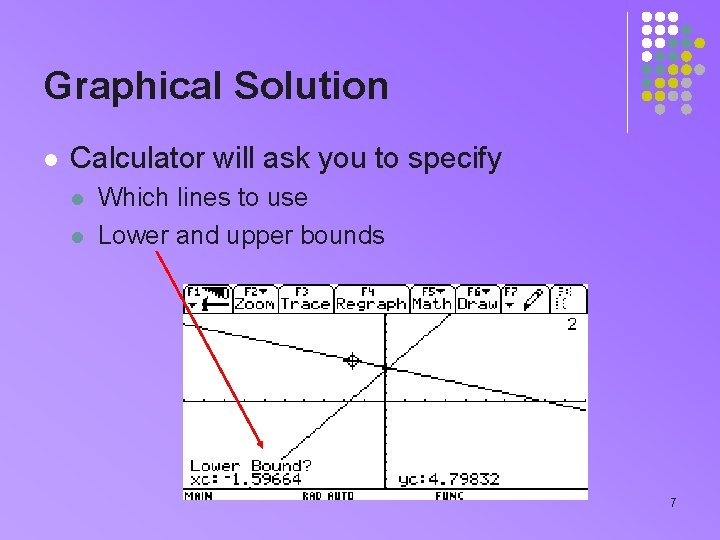Graphical Solution l Calculator will ask you to specify l l Which lines to use Lower and upper bounds 7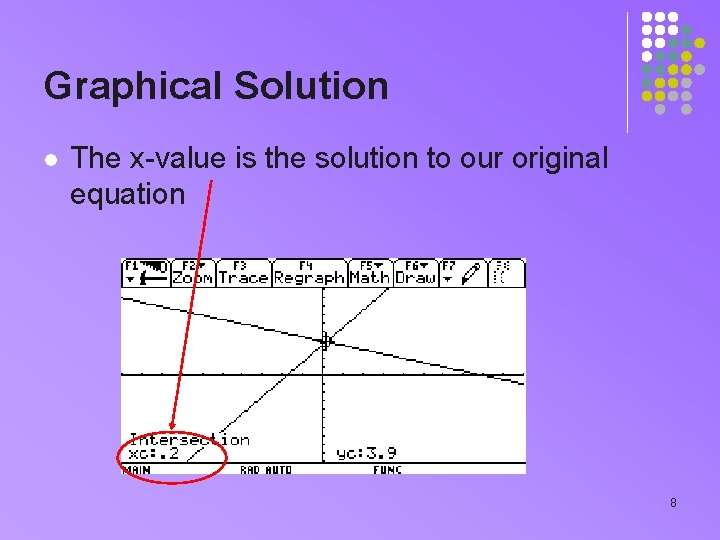Graphical Solution l The x-value is the solution to our original equation 8Numeric Solution l Given l l l Let each side be a function Place in Y= screen of calculator View table ♦Y, l l l Adjust starting point, increment Narrow down search, adjust again Look for matches 9Assignment A l l l Lesson 2. 3 A Page 103 Exercises 1 – 49 odd 10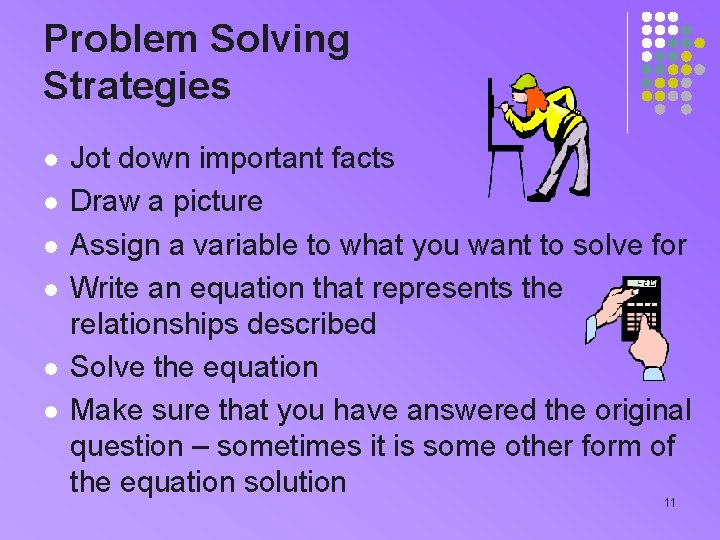Problem Solving Strategies l l l Jot down important facts Draw a picture Assign a variable to what you want to solve for Write an equation that represents the relationships described Solve the equation Make sure that you have answered the original question – sometimes it is some other form of the equation solution 11Example l A person 66 inches tall is standing 15 ft from a streetlight. If the person casts a shadow 84 inches long, how tall is the streetlight?Another Example l At 2: 00 a runner heads north on a highway jogging at 10 mph. At 2: 30 a driver heads north on the same highway to pick up the runner. The car was traveling at 55 mph. How long will it take the driver to catch the runner? Remember that Dist = Rate * Time Hint: What two things are equal so we can write an equation? 13Assignment B l l l Lesson 2. 3 B Page 104 Exercises 53, 55, 57, 59, 63, 65, 67, 69, 71 14